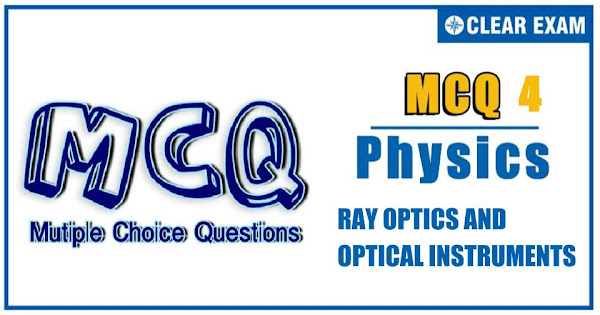## [LATEST]\$type=sticky\$show=home\$rm=0\$va=0\$count=4\$va=0

JEE Advanced Physics Syllabus can be referred by the IIT aspirants to get a detailed list of all topics that are important in cracking the entrance examination. JEE Advanced syllabus for Physics has been designed in such a way that it offers very practical and application-based learning to further make it easier for students to understand every concept or topic by correlating it with day-to-day experiences. In comparison to the other two subjects, the syllabus of JEE Advanced for physics is developed in such a way so as to test the deep understanding and application of concepts.

Q1. A plano-convex glass lens (μ_g=3/2) of radius of curvature R=10 cm is placed at a distance of ‘b’ from a concave lens of focal length 20 cm
•  40 cm
•  60 cm
•  30 cm
•  20 cm
Solution
Focal length of plano convex lens is 1/f=(3/2-1)(1/10-1/∞)or f=20 cm If point object O is placed at a distance of 20 cm from the plano-convex lens, rays become parallel and final image is formed at second focus or 20 cm from concave lens which is independent of b
Q2.A thin equiconvex lens (μ=3/2) of focal length 10 cm is cut and separated and a material of refractive index 3 is filled between them. What is the focal length of the combination?

•  -10 cm
•  -10/4 cm
•  -10/3 cm
•  None of these
Q3.  A concave mirror of focal length 10 cm and a convex mirror of focal length 15 cm are placed facing each other 40 cm apart. A point object is placed between the mirrors, on their common axis and 15 cm from the concave mirror. Find the position and nature of the image produced by successive reflections, first at the concave mirror and then at the convex mirror
•   12 cm behind convex mirror, real
•  9 cm behind convex mirror, real
•  6 cm behind convex mirror, virtual
•  3 cm behind convex mirror, virtual

Q4. A cubical block of glass, refractive index 1.5, has a spherical cavity of radius r=9 cm inside it as shown in figure. A luminous point object O is at a distance of 18 cm from the cube (see figure). What is the apparent position of O as seen from A?
•  μ>3/4
•  μ less than (3√3)/4
•  μ>√3
•  μ less than √3/2
Q5. An object is approaching a fixed plane mirror with velocity 5 ms^(-1) making an angle of 45° with the normal. The speed of image w.r.t. the mirror is
•  5 ms^(-1)
•  5/√2 ms^(-1)
•  5√2 ms^(-1)
•  10 ms^(-1)
Q6. Behind a thin converging lens having both the surfaces of the same radius 1 cm, a plane mirror has been placed. The image of an object at a distance of 40 cm from the lens is formed at the same position. What is the refractive index of the lens?
•  1.5
•  5/3
• 9/8
•  None of these
Q7.In an experiment to determine the focal length (f) of a concave mirror by the u-v method, a student places the object pin Aon the principle axis at a distance x from the pole P. The student looks at the pin and its inverted image from a distance keeping his/her eye in line with PA. When the student shifts his/her eye towards left, the image appears to the right of the object pin. Then
•  x less than f
•  f less than x less than 2f
•  x=2f
•  x>2f
Q8.A ray of light is incident on a medium with angle of incidence i and refracted into a second medium with angle of refraction r. The graph of sin⁡〖(i)〗 vs sin⁡〖(r)〗 is as shown in figure. Then, the velocity of light in the first medium in n times the velocity of light in the second medium. What should be the value of n?
•  √3
•  T1/√3
•  √3/2
•  2/√3
Solution

From Snell’s law, μ_1 sin⁡i=μ_2 sin⁡r ⇒μ_2/m_1 =sin⁡i/sin⁡r From the graph, tan⁡〖60°〗=sin⁡i/sin⁡r ⇒sin⁡i/sin⁡r =√3=μ_2/μ_1 μ=c/v⇒ =μ_1/μ_2 =v_2/v_1 v_1=(μ_2/μ_1 ) v_2 ⇒v_1=√3 v_2

Q9.A candle placed 20 cm from the surface of a convex mirror and a plane mirror is also placed so that the virtual images in the two mirrors coincide. If the plane mirror is 12 cm away from the object, what is the focal length of the convex mirror?
•  20 cm
•  15 cm
•  10 cm
•  5 cm
Solution

For convex mirror, u+v=12×2=24 cm (because for plane mirror, distance of object=distance of image). Also, here for the convex mirror u=20 cm, therefore v=4 cm. Hence, using 1/v+1/u=1/f We find f=5 cm
Q10. The given lens is broken into four parts rearranged as shown. If the initial focal length is f, then after rearrangement the equivalent focal length is
•  4.5 ms^(-1)
•  5.4 ms^(-1)
•  3.0 ms^(-1)
• 3.4 ms^(-1)#### Written by: AUTHORNAME

AUTHORDESCRIPTION## Want to know more

Please fill in the details below:

## Latest NEET Articles\$type=three\$c=3\$author=hide\$comment=hide\$rm=hide\$date=hide\$snippet=hide

Name

ltr
item
BEST NEET COACHING CENTER | BEST IIT JEE COACHING INSTITUTE | BEST NEET & IIT JEE COACHING: Ray Optics and Optical Instruments-Quiz-4
Ray Optics and Optical Instruments-Quiz-4
https://1.bp.blogspot.com/-JMW5vVwG8fM/YN3Bo2OTHHI/AAAAAAAALs0/obV_G3ml6qIKp-xZu-uNeRMiNuUD_sTmQCLcBGAsYHQ/s600/Quiz%2BImage%2B20%2B%25281%2529.jpg
https://1.bp.blogspot.com/-JMW5vVwG8fM/YN3Bo2OTHHI/AAAAAAAALs0/obV_G3ml6qIKp-xZu-uNeRMiNuUD_sTmQCLcBGAsYHQ/s72-c/Quiz%2BImage%2B20%2B%25281%2529.jpg
BEST NEET COACHING CENTER | BEST IIT JEE COACHING INSTITUTE | BEST NEET & IIT JEE COACHING
https://www.cleariitmedical.com/2021/07/ray-optics-and-optical-instruments-quiz_5.html
https://www.cleariitmedical.com/
https://www.cleariitmedical.com/
https://www.cleariitmedical.com/2021/07/ray-optics-and-optical-instruments-quiz_5.html
true
7783647550433378923
UTF-8

STAY CONNECTED